# Solved 2022 Semester 2 Question Paper ICSE Class 10 Chemistry

## SECTION A (10 Marks)

#### Question 1(i)

The ore of Aluminium is:

1. Calamine
2. Haematite
3. Magnetite
4. Cryolite

Cryolite

Reason — Cryolite [Na3AlF6] is an ore of aluminium. Calamine [ZnCO3], Haematite [Fe2O3], Magnetite [Fe3O4] are not ores of aluminium.

#### Question 1(ii)

Hydrogen chloride gas is not collected over water, as:

1. It is highly soluble in water.
2. It is less soluble in water.
3. It is lighter than air.
4. It is heavier than air.

It is highly soluble in water

Reason — Hydrogen chloride gas is not collected over water since it is highly soluble in water.

#### Question 1(iii)

An aqueous solution of ammonia is:

1. Neutral
2. Acidic
3. Basic
4. Amphoteric

Basic

Reason — The aq. soln. of ammonia [NH4OH] is basic in nature.

#### Question 1(iv)

The acid which is least volatile is:

1. Hydrochloric acid
2. Nitric acid
3. Dilute sulphuric acid
4. Concentrated sulphuric acid

Concentrated Sulphuric acid

Reason — Concentrated sulphuric acid has a high boiling point so it considered to be a non-volatile acid.

#### Question 1(v)

The gas formed, when calcium bisulphite reacts with dilute HNO3:

1. Sulphur trioxide
2. Hydrogen
3. Sulphur dioxide
4. Hydrogen sulphide

Sulphur dioxide

Reason — Ca(HSO3)2 + 2HNO3 [dil.] ⟶ Ca(NO3)2 + 2H2O + 2SO2

#### Question 1(vi)

The IUPAC name of formic acid:

1. Propanoic acid
2. Methanoic acid
3. Ethanoic acid
4. Butanoic acid

Methanoic acid

Reason — The IUPAC name of formic acid [HCOOH] is methanoic acid.

#### Question 1(vii)

The metallic oxide which when reacts with HCl forms salt and water:

1. Carbon monoxide
2. Nitrous oxide
3. Ammonium hydroxide
4. Sodium oxide

Sodium oxide

Reason — Sodium oxide reacts with HCl and forms the salt sodium chloride and water

2HCl + Na2O ⟶ 2NaCl + H2O

#### Question 1(viii)

Vanadium pentoxide is used as a catalyst in the preparation of:

1. Nitrogen gas
2. Nitrogen dioxide gas
3. Sulphur trioxide gas
4. Carbon dioxide gas

Sulphur trioxide gas

Reason — Vanadium pentoxide is used during contact process for the preparation of sulphur trioxide gas.

$2\text{SO}_2 + \text{O}_2 \xrightleftharpoons [450-500\text{\degree C }/1 - 2 \text{ atmos}]{\text{V}_2\text{O}_5} 2\text{SO}_3 + \text{45 K cals}$

#### Question 1(ix)

The catalyst used for the conversion of ethene to ethane:

1. Iron
2. Nickel
3. Cobalt
4. Molybdenum

Nickel

Reason — The catalyst used for conversion of ethene to ethane is commonly nickel.

$\underset{\text{ ethene}}{\text{C}_2\text{H}_4} + \text{H}_2 \xrightarrow[300\degree\text{C}]{\text{Nickle}} \underset{\text{ethane}}{\text{C}_2\text{H}_6}$

#### Question 1(x)

Substance which helps to lower the fusion point of the mixture in Hall Heroult Process:

1. Coke
2. Concentrated sodium hydroxide
3. Fluorspar
4. Concentrated potassium hydroxide

Fluorspar

Reason — Fluorspar lowers the fusion point of the mixture in Hall Heroult Process and the mixture fuses at 950° C instead of 2050° C.

## SECTION B (30 Marks)

#### Question 2(i)

Define:

(a) Isomerism

(b) Ores

(a) Isomerism — Isomerism is the phenomenon due to which two or more compounds have the same molecular formula but differ in molecular arrangement or in structural formula.

(b) Ores — Ores are minerals from which metals are extracted commercially at a comparatively lower cost and with minimum effort.

#### Question 2(ii)

Name the following:

(a) The property by which carbon links with itself to form a long chain.

(b) The saturated hydrocarbons having general formula CnH2n-2.

(a) Catenation

(b) Alkyne

#### Question 2(iii)

Draw the structural diagram of:

(a) pentanal

(b) propanol

(c) 2-butene

(a) pentanal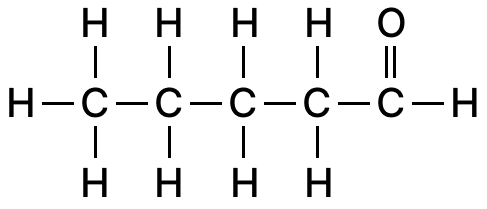(b) propanol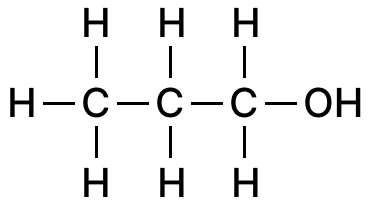(c) 2-butene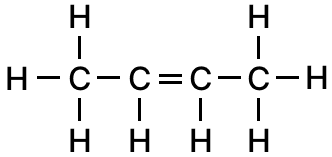#### Question 2(iv)

Complete and balance the following chemical equations:

(a) H2C = CH2 + Cl2

(b) C2H6 + O2 [excess] ⟶

(c) CH4 + O2 [excess] ⟶

(a) H2C = CH2 + Cl2 ⟶ CH2Cl – CH2Cl

(b) 2C2H6 + 7O2 [excess] ⟶ 4CO2 + 6H2O

(c) CH4 + 2O2 [excess] ⟶ CO2 + 2H2O

#### Question 3(i)

State the following:

(a) A compound formed when excess ammonia gas reacts with chlorine.

(b) A substance added to water, to manufacture sulphuric acid in Contact process.

(a) Ammonium chloride [NH4Cl]

(b) Oleum

#### Question 3(ii)

Identify the gas P and Q in the reactions given below:

(a) A compound reacts with an acid to form gas P which has no effect on acidified K2Cr2O7 solution but turns lime water milky.

(b) A metallic nitrate reacts on heating gives oxygen gas along with a coloured gas Q.

(a) Carbon dioxide [CO2]

(b) Nitrogen dioxide [NO2]

#### Question 3(iii)

State the observation for the following:

(a) Dry ammonia gas reacts with oxygen in the presence of a catalyst.

(b) Excess chlorine gas reacts with ammonia gas.

(c) Carbon reacts with hot concentrated nitric acid.

(a) Dry ammonia gas reacts with oxygen in the presence of platinum (catalyst) to produce nitric oxide (NO), water and heat. Nitric oxide (NO) produced is oxidised to nitrogen dioxide (NO2) and reddish brown vapours are seen in the flask. As the reaction is exothermic, so the platinum (catalyst) continues to glow even after the heating is discontinued.
4NH3 + 5O2 $\xrightarrow[800 \degree \text{C}]{\text{Pt.}}$ 6H2O + 4NO↑ + Δ
2NO + O2 ⟶ 2NO2

(b) Colourless ammonia gas reacts with greenish yellow chlorine gas in excess producing hydrogen chloride and a yellow explosive liquid (nitrogen trichloride).
NH3 + 3Cl2 [excess] ⟶ 3HCl + NCl3

(c) Carbon reacts with hot concentrated nitric acid producing carbon dioxide, water and reddish brown fumes of nitrogen dioxide are evolved.
C + 4HNO3 ⟶ CO2 + 2H2O + 4NO2

#### Question 3(iv)

Write balanced equation for the following conversions:

(a) Carbon from cane sugar and concentrated sulphuric acid.

(b) Ferric nitrate from ferric hydroxide and nitric acid.

(c) Ammonium sulphate from ammonium hydroxide and sulphuric acid.

(a) C12H22O11 $\xrightarrow{\text{Conc. H}_2\text{SO}_4}$ 12C (sugar charcoal) + 11H2O

(b) Fe(OH)3 + 3HNO3 [dil.] ⟶ Fe(NO3)3 + 3H2O

(c) 2NH4OH + H2SO4 ⟶ (NH4)2SO4 + 2H2O

#### Question 4(i)

State the relevant reason for the following:

(a) Concentrated alkali is used for the concentration of bauxite ore.

(b) Fused alumina is reduced to aluminium by electrolysis.

(a) Bauxite is heated under pressure with conc. caustic soda (NaOH) for 2 to 8 hours. Bauxite dissolves and forms sodium meta aluminate because of the amphoteric nature of aluminium, leaving behind insoluble impurities called red mud. Red mud consists of ferric oxide, sand, etc. which are removed by filtration.

(b) Aluminium oxide due to its great affinity for oxygen is a very stable compound. It is not reduced easily by common reducing agents like carbon, carbon monoxide and hydrogen. Hence, fused alumina can be reduced to aluminium only by electrolysis.

#### Question 4(ii)

State one use of the given alloys:

(a) Magnalium

(b) Duralumin

(a) Magnalium is used for making aircraft parts and scientific instruments.

(b) Duralumin is used for making light tools and pressure cooker.

#### Question 4(iii)

Complete the table given below which refers to the Laboratory preparation of Ammonia gas:

Laboratory preparationReactants usedProducts formedDrying agentMethod of collection
Ammonia gas(a) ...............Calcium chloride + water + ammonia(b) ...............(c) ...............

Laboratory preparationReactants usedProducts formedDrying agentMethod of collection
Ammonia gas(a) ammonium chloride [NH4Cl] and calcium hydroxide [Ca(OH)2)]Calcium chloride + water + ammonia(b) quicklime [CaO](c) downward displacement of air

#### Question 4(iv)

Identify the terms for the following:

(a) The process used to purify Alumina by electrolytic reduction.

(b) The experiment used to demonstrate the high solubility of HCl gas.

(c) The chemical property of sulphuric acid to form two types of salts with an alkali.

(a) Hall-Heroult's process

(b) Fountain experiment

(c) Dibasic property of sulphuric acid.

#### Question 5(i)

Write the balanced chemical equation for the following:

(a) Action of heat on manganese dioxide and concentrated hydrochloric acid.

(b) Zinc reacts with dilute hydrochloric acid to form zinc chloride.

(a) MnO2 + 4HCl ⟶ MnCl2 + 2H2O + Cl2

(b) Zn + 2HCl ⟶ ZnCl2 + H2

#### Question 5(ii)

Select the right answer from the brackets and complete the statements:

In electrolysis of fused Alumina, the anode is made of (a) ............... [gas carbon/graphite] and the product formed at cathode is (b) ............... [oxygen/aluminium].

In electrolysis of fused Alumina, the anode is made of (a) graphite and the product formed at cathode is (b) aluminium

#### Question 5(iii)

Give the IUPAC name for the following: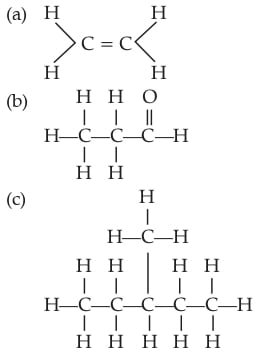(a) Ethene

(b) Propanaldehyde

(c) 3-methyl Pentane

#### Question 5(iv)

Study the diagram, which shows the Brown Ring Test and answer the questions given below: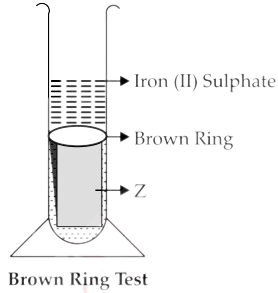(a) Which ion is determined by Brown Ring Test?

(b) Why is freshly prepared iron[II] sulphate used in the test?

(c) Name the substance Z.

(a) Nitrate ion [NO31-]

(b) As iron [II] sulphate on exposure to the atmosphere is oxidised to iron [III] sulphate and the test will not answer with iron [III] sulphate, hence, freshly prepared iron[II] sulphate used in the test.

(c) Concentrated Sulphuric Acid.

#### Question 6(i)

Distinguish between the following as directed:

(a) Sodium sulphite solution and sodium sulphate solution. [using dilute H2SO4]

(b) Lead salt solution and zinc salt solution. [using NH4OH solution in excess]

(a) On adding dilute sulphuric acid to sodium sulphite solution and warming, a colourless gas with suffocating smell of burning sulphur is evolved. The evolved gas turns lime water milky and changes the colour of acidified K2Cr2O7 solution from orange to green suggesting that it is SO2.

Na2SO3 + H2SO4 (dil.) ⟶ Na2SO4 + H2O + SO2

Sodium sulphate does not react with dilute sulphuric acid as an acid does not react with its own salt due to the presence of same anion.

(b) A lead salt gives a chalky white ppt. on reaction with ammonium hydroxide that is insoluble in excess of ammonium hydroxide. For example:

Pb(NO3)2 + 2NH4OH ⟶ 2NH4NO3 + Pb(OH)2

On the other hand, zinc salt forms a white gelatinous ppt. which dissolves when excess of ammonium hydroxide is added. Hence, the two can be distinguished.

ZnSO4 + 2NH4OH ⟶ (NH4)2SO4 + Zn(OH)2

Zn(OH)2 + (NH4)2SO4 + 2NH4OH ⟶ [Zn(NH3)4]SO4 + 4H2O

#### Question 6(ii)

Give one word for the following statements:

(a) The compounds of various metals found in nature with earthly impurities.

(b) A homogeneous mixture of two or more metals or a metal and a non-metal in specific ratios.

(a) Minerals

(b) Alloys

#### Question 6(iii)

Identify the acid in each case:

(a) The acid formed when sulphur reacts with concentrated nitric acid.

(b) An acid, which on adding to lead nitrate solution produces a white precipitate which is soluble on heating.

(c) The acid formed when potassium nitrate reacts with a least volatile acid.

(a) Sulphuric acid.

(b) Hydrochloric acid

(c) Nitric acid

#### Question 6(iv)

Match column A with column B:

Name (A)Functional group (B)
1. Aldehyde(a) —OH
2. Carboxylic acids(b) —CHO
3. Alcohol(c) —COOH